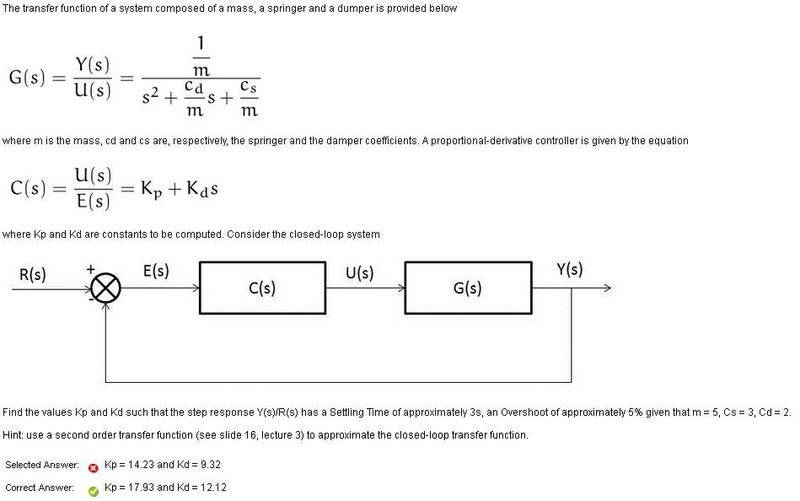# 2nd Order Control system PD controller

Hi,

This question on PD control is from a practice quiz.

1. Homework StatementIf you can't see it- the question asks to find values for Kp and Kd such that the system achieves 5% OS and has a settling time Ts of 3s.
Cs = 3
Cd = 2
m = 5

## Homework Equations

ω_n^2/(s^2 + 2ζw_n + ω_n^2) - 2nd order form

Overall Transfer Function I found:
Y/R = CG/1+CG = [ (Kp + Kds)/m ] / [ s^2 + ((Cd + Kd)/m) + ((Cs + Kp)/m)]

Ts = 4/σ (2% of final value); where σ = ζω_n

## The Attempt at a Solution

From the transfer function I found above i noticed that it is not exactly in the 2nd order form. However I was told that we still set

ω_n = SQRT[ ((Cs + Kp)/m) ] {1}
because when we add a derivative controller it only adds a zero which effects the numerator, not the poles on the denominator.

firstly we know for 5%OS → ζ≈0.7
from Ts = 3 = 4/ζω_n
→ ω_n = 4/3ζ = 40/21

from eqn {1} - solving for Kp
Kp = (ω_n^2)m - Cs = (5)(40/21)^2 - 3 = 15.14

At this point I stopped because their answers were Kp = 17.93 and Kd = 12.12.
Could you explain if the process i used is incorrect because I cant understand what I am doing wrong.

Thank you
(btw I just joined Physics Forums like an hour ago)

#### Attachments

donpacino
Gold Member
There are often many ways to solve control problems. Plot your solution. Does it meet the design criteria?

hi, can someone please have a look at my working to see if im on the right track, I spent many hours on it and im not even sure if this is the correct way.

donpacino, Im a beginner in Matlab, Im not sure how a plot would verify my results.

donpacino
Gold Member
hi, can someone please have a look at my working to see if im on the right track, I spent many hours on it and im not even sure if this is the correct way.

donpacino, Im a beginner in Matlab, Im not sure how a plot would verify my results.
run a simulation to see if your KP and KI values meet the criteria for rise time and settling time.

There are often multiple solutions that will work for problems like this